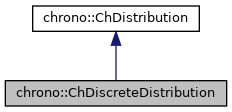chrono::ChDiscreteDistribution Class Reference

## Description

Class that can be used to generate sample numbers according to a discrete probability distribution.

Probability distribution is defined with discrete values, each with a percentual of probability.

#include <ChDistribution.h>

Inheritance diagram for chrono::ChDiscreteDistribution:[legend]
Collaboration diagram for chrono::ChDiscreteDistribution:[legend]

## Public Member Functions

ChDiscreteDistribution (ChVectorDynamic<> &mx, ChVectorDynamic<> &my)
Create an object that can be used to generate sample numbers according to a discrete probability distribution. More...

virtual double GetRandom () override
Compute a random value, according to the discrete probability values entered when you created this object.

const ChVectorDynamicGetProbabilityXpoints () const

const ChVectorDynamicGetProbabilityYpoints () const

const ChVectorDynamicGetProbabilityCDFcumulativeY () constPublic Member Functions inherited from chrono::ChDistribution
virtual ~ChDistribution ()
Default destructor for distribution object.

## ◆ ChDiscreteDistribution()

 chrono::ChDiscreteDistribution::ChDiscreteDistribution ( ChVectorDynamic<> & mx, ChVectorDynamic<> & my )

Create an object that can be used to generate sample numbers according to a discrete probability distribution.

Probability distribution is defined with N discrete values, each with a percentual of probability. The sum of the N probability values must be unit, i.e normalized (but if not, a normalization will be enforced) For example, to get '12.3' for 30% of the times you call GetRandom(), and '150' for the remaining 70% of the times, create ChDiscreteDistribution with mx = [12.3; 150] and my = [0.3; 0.7]

The documentation for this class was generated from the following files:
• /builds/uwsbel/chrono/src/chrono/core/ChDistribution.h
• /builds/uwsbel/chrono/src/chrono/core/ChDistribution.cpp